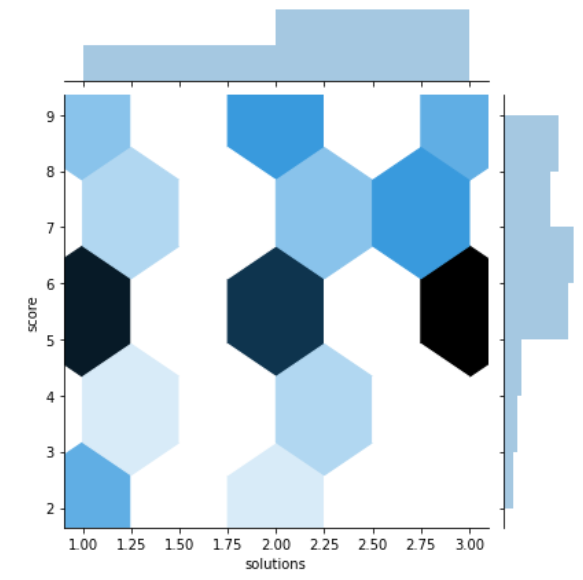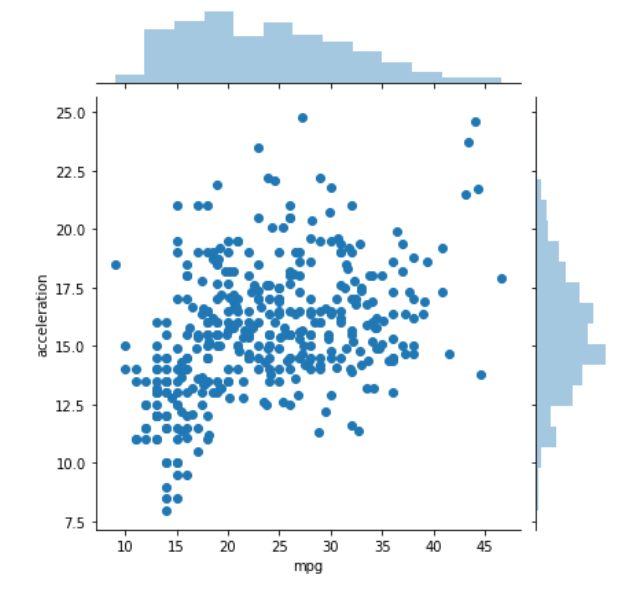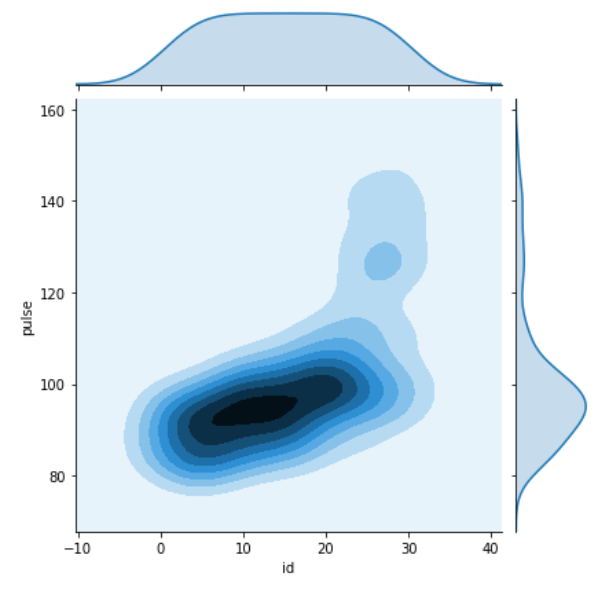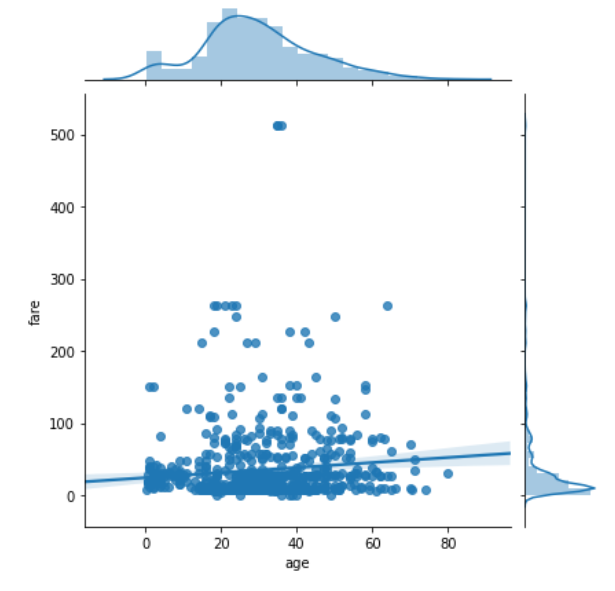# Python – seaborn.jointplot() method

• Last Updated : 01 Aug, 2020

Seaborn is a Python data visualization library based on matplotlib. It provides a high-level interface for drawing attractive and informative statistical graphics. Seaborn helps resolve the two major problems faced by Matplotlib; the problems are ?

• Default Matplotlib parameters
• Working with data frames

As Seaborn compliments and extends Matplotlib, the learning curve is quite gradual. If you know Matplotlib, you are already half-way through Seaborn.

Attention geek! Strengthen your foundations with the Python Programming Foundation Course and learn the basics.

To begin with, your interview preparations Enhance your Data Structures concepts with the Python DS Course. And to begin with your Machine Learning Journey, join the Machine Learning - Basic Level Course

### seaborn.jointplot() :

Draw a plot of two variables with bivariate and univariate graphs. This function provides a convenient interface to the ‘JointGrid’ class, with several canned plot kinds. This is intended to be a fairly lightweight wrapper; if you need more flexibility, you should use :class:’JointGrid’ directly.

Syntax: seaborn.jointplot(x,  y,  data=None, kind=’scatter’, stat_func=None, color=None, height=6, ratio=5, space=0.2, dropna=True,  xlim=None, ylim=None, joint_kws=None, marginal_kws=None, annot_kws=None, **kwargs)

Parameters: The description of some main parameters are given below:

x, y: These parameters take Data or names of variables in “data”.

data: (optional) This parameter take DataFrame when “x” and “y” are variable names.

kind: (optional) This parameter take Kind of plot to draw.

color:  (optional) This parameter take Color used for the plot elements.

dropna: (optional) This parameter take boolean value, If True, remove observations that are missing from “x” and “y”.

Return: jointgrid object with the plot on it.

Below is the implementation of above method:

Example 1:

## Python3

 `# importing required packages``import` `seaborn as sns``import` `matplotlib.pyplot as plt`` ` `# loading dataset``data ``=` `sns.load_dataset(``"attention"``)`` ` `# draw jointplot with``# hex kind``sns.jointplot(x ``=` `"solutions"``, y ``=` `"score"``,``              ``kind ``=` `"hex"``, data ``=` `data)``# show the plot``plt.show()`` ` `# This code is contributed ``# by Deepanshu Rustagi.`

Output:Example 2:

## Python3

 `# importing required packages``import` `seaborn as sns``import` `matplotlib.pyplot as plt`` ` `# loading dataset``data ``=` `sns.load_dataset(``"mpg"``)`` ` `# draw jointplot with``# scatter kind``sns.jointplot(x ``=` `"mpg"``, y ``=` `"acceleration"``,``              ``kind ``=` `"scatter"``, data ``=` `data)``# show the plot``plt.show()`` ` `# This code is contributed``# by Deepanshu Rustagi.`

Output:Example 3:

## Python3

 `# importing required packages``import` `seaborn as sns``import` `matplotlib.pyplot as plt`` ` `# loading dataset``data ``=` `sns.load_dataset(``"exercise"``)`` ` `# draw jointplot with``# kde kind``sns.jointplot(x ``=` `"id"``, y ``=` `"pulse"``,``              ``kind ``=` `"kde"``, data ``=` `data)``# Show the plot``plt.show()`` ` `# This code is contributed``# by Deepanshu Rustagi.`

Output:Example 4:

## Python3

 `# importing required packages``import` `seaborn as sns``import` `matplotlib.pyplot as plt`` ` `# loading dataset``data ``=` `sns.load_dataset(``"titanic"``)`` ` `# draw jointplot with``# reg kind``sns.jointplot(x ``=` `"age"``, y ``=` `"fare"``,``              ``kind ``=` `"reg"``, data ``=` `data,``              ``dropna ``=` `True``)`` ` `# show the plot``plt.show()`` ` `# This code is contributed ``# by Deepanshu Rustagi.`

Output:My Personal Notes arrow_drop_up